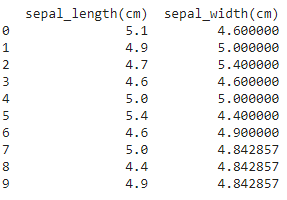# How to Fix: ValueError: All arrays must be of the same length

• Last Updated : 28 Nov, 2021

In this article we will fix the error: All arrays must be of the same length. We get this error when we create a pandas data frame with columns of different lengths but when we are creating pandas dataframe the columns should be equal instead there can be NaN in the deficient cell of the column.

Error:

`ValueError: All arrays must be of the same length`

## Python3

 `# import pandas module``import` `pandas as pd`` ` ` ` `# consider the lists``sepal_length ``=` `[``5.1``, ``4.9``, ``4.7``, ``4.6``, ``5.0``, ``5.4``, ``                ``4.6``, ``5.0``, ``4.4``, ``4.9``]``sepal_width ``=` `[``4.6``, ``5.0``, ``5.4``, ``4.6``, ``5.0``, ``4.4``, ``4.9``]`` ` `# DataFrame with two columns``df ``=` `pd.DataFrame({``'sepal_length(cm)'``: sepal_length,``                   ``'sepal_width(cm)'``: sepal_width})``# display``print``(df)`

Output:

`ValueError: arrays must all be same length`

### Reason for the error :

The length of the list sepal_length which is going to be the column was not equal to length of the list sepal_witdth column.

`len(sepal_length)!= len(sepal_width)`

## Fixing the error:

The error can be fixed by adding the values to the deficient list or deleting the list with a larger length if it has some useless values. NaN or any other value can be added to the deficient value based on the observation of the remaining values in the list.

Syntax:

Considering two lists list1 and list2:

```if (len(list1) > len(list2)):
list2 += (len(list1)-len(list2)) * [any_suitable_value]
elif (len(list1) < len(list2)):
list1 += (len(list2)-len(list1)) * [any_suitable_value]```

Here, any_suitable_value can be an average of the list or 0 or NaN based on the requirement.

Example:

## Python3

 `# importing pandas``import` `pandas as pd``# importing statistics``import` `statistics as st`` ` `# consider the lists``sepal_length ``=` `[``5.1``, ``4.9``, ``4.7``, ``4.6``, ``5.0``, ``5.4``,``                ``4.6``, ``5.0``, ``4.4``, ``4.9``]``sepal_width ``=` `[``4.6``, ``5.0``, ``5.4``, ``4.6``, ``5.0``, ``4.4``, ``4.9``]`` ` ` ` `# if length are not equal``if` `len``(sepal_length) !``=` `len``(sepal_width):``    ``# Append mean values to the list with smaller length``    ``if` `len``(sepal_length) > ``len``(sepal_width):``        ``mean_width ``=` `st.mean(sepal_width)``        ``sepal_width ``+``=` `(``len``(sepal_length)``-``len``(sepal_width)) ``*` `[mean_width]``    ``elif` `len``(sepal_length) < ``len``(sepal_width):``        ``mean_length ``=` `st.mean(sepal_length)``        ``sepal_length ``+``=` `(``len``(sepal_width)``-``len``(sepal_length)) ``*` `[mean_length]`` ` ` ` `# DataFrame with 2 columns``df ``=` `pd.DataFrame({``'sepal_length(cm)'``: sepal_length,``                   ``'sepal_width(cm)'``: sepal_width})``print``(df)`

Output:My Personal Notes arrow_drop_up